# Subtraction Worksheets for Grade 3Q.1) Subtract the following: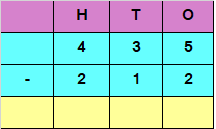a) 223​
b) ​323
c) 231​

Q.2) Subtract the following:a) 6454​
b) ​7024
c) ​7464

Q.3) Subtract the following: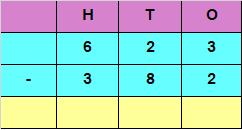a) 191​
b) ​241
c) ​245

Q.4) Subtract the following: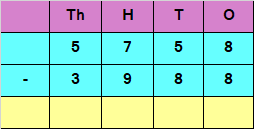a) 1490​
b) ​1770
c) ​1320

### Subtraction Worksheets for Grade 3 Explanations

Q.1) Explanation –

Subtracting the digits at ones place
5 – 2 = 3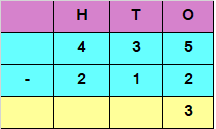Subtracting the digits at tens place
3 – 1 = 2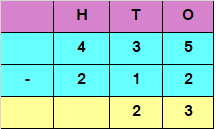Subtracting the digits at hundreds place

4 – 2 = 2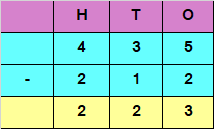Hence, the difference is 223

Q.2) Explanation –

Subtracting the digits at ones place
8 – 4 = 4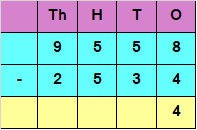Subtracting the digits at tens place

5 – 3 = 2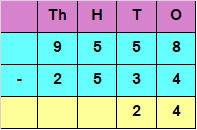Subtracting the digits at hundreds place

5 – 5 = 0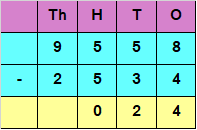Subtracting the digits at thousands place

9 – 2 = 7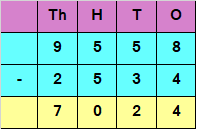Hence, the difference is 7024

Q.3) Explanation –

Subtracting the digits at ones place

3 – 2 = 1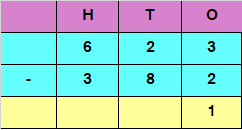If we want to Subtract the digits at tens place, we have

2 – 8

But 8 is greater than 2

We would need to borrow 1 from 6 in hundreds place

2 in tens place would now become 12 and 6 in hundreds place would be reduced to 5

So, 12 – 8 = 4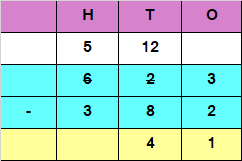Subtracting the digits at hundreds place

5 – 3 = 2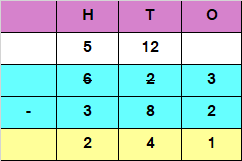Hence, the difference is 241

Q.4) Explanation –

Subtracting the digits at ones place
8 – 8 = 0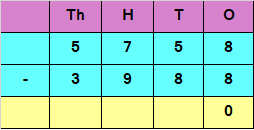If we want to Subtract the digits at tens place, we have

5 – 8

But 8 is greater than 5

We would need to borrow 1 from 7 in hundred place

5 in ones place would now become 15 and 7 in hundred place would be reduced to 6

So, 15 – 8 = 7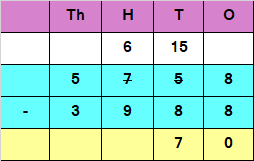If we want to Subtract the digits at hundred place, we have

6 – 9

But 9 is greater than 6

We would need to borrow 1 from 5 in thousand place

6 in hundred place would now become 16 and 5 in thousand place would be reduced to 4

So, 16 – 9 = 7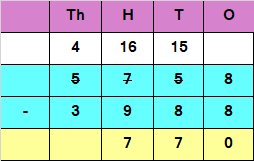Subtracting the digits at thousands place

4 – 3 = 1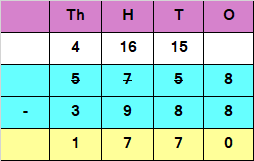Hence, the difference is 1770